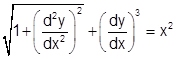×#### Thank you for registering.

One of our academic counsellors will contact you within 1 working day.

Click to Chat

1800-1023-196

+91-120-4616500

CART 0

• 0

MY CART (5)

Use Coupon: CART20 and get 20% off on all online Study Material

ITEM
DETAILS
MRP
DISCOUNT
FINAL PRICE
Total Price: Rs.

There are no items in this cart.
Continue Shopping```Basic Definition of Differential EquationA differential equation is an equation which involves an unknown function of one or several variables that relates the values of the function itself and its derivatives of various orders. Differential equations play a vital role in various disciplines besides mathematics like engineering, physics, economics and biology.

In other words, a differential equation may be defined as an equation containing an independent variable, dependent variable and differential coefficients of dependent variable with respect to independent variable.

For Example: Listed below are the various examples of differential equations:

xdy/dx =3y                               ......... (1)......... (2)

√dy/dx + y = x                  .......... (3)......... (4)

(d2y/dx2 )3 + (dy/dx)2 = x                 ......... (5)......... (6)

Order and Degree of a Differential Equation:

The order of differential equation is the order of highest order derivative appearing in the equation. So an equation of order 1 is called a first order equation, of order 2 is called a second order equation.

For Example :

Orders of differential equations (1), (2), (3), (4), (5) and (6) are 1, 2, 1, 3, 2 and 2 respectively.

The differential equation y" + xy' – x3y = sin x is of second order since the highest derivative is y".

The degree of differential equation is the degree of the highest order derivative involved in it, when the differential coefficients are free from radicals and fractions (i.e. write differential equations as polynomial in derivatives)

For Example:

Degrees of differential equations (1), (2), (3), (4), (5) and (6) are 1, 1, 1, 4, 3 and 2 respectively.

For more on differential equations, you may refer the video

Illustration 1: Find the order and degree (if defined) of the following differential equations:

(i) (d3y/dx3 )2/3 = dy/dx +2.

(ii)  d2y/dx2 = x (dy/dx)

Solution :

(i) The given differential equation can be re-written as

(d3y/dx3 )2/3 = dy/dx +2.

(d3y/dx3 )2= (dy/dx +2)3

Hence its order is 3 and degree 2.

(ii) d2y/dx2 = x (dy/dx)

This is already in the standard form. Hence its order is 2 and degree 1.

The differential equations can be classified into the ordinary or the partial differential equations:

Ordinary Differential Equations: An ordinary differential equation is an equation involving a function and its derivatives. In other words, an equation involving one independent variable is called an ordinary differential equation. Mathematically, an Ordinary differential equation also called the ODE is expressed as

F(x, y, y',…..,y (n) ) = 0, where y is a function of x and y' denotes the first derivative of y with respect to x, and y(n) = dny / dxn

An ordinary differential equation of order n is said to be linear if

an (x)y(n) + a n-1(x) y (n-1) + …. + a1 (x) y' + a0 (x) y = Q(x)

Further, if in such an equation Q(x) = 0, then the equation is said to be homogeneous.

Partial Differential Equations: A partial differential equation (PDE) is an equation involving functions and their partial derivatives. An example of a partial differential equation isIllustration: Solve the ODE:

dx/dt = 5x -3

Solution: It is a very simple equation. First bring the terms of x on one side and then attempt to solve.

So, we get dx / 5x-3 = dt.

Integrating both sides, we obtain

1/5 log |5x-3| = t + c

Though this is the answer but it can be further simplified.

(5x-3) = ±exp(5t + c)

x= ±1/5 exp(5t + c) +3/5

There are various methods of solving differential equations but they vary according to the type of equation. The various types of equations and their methods have been discussed in detail in the coming sections. Differential Equations is the most scoring portion of the JEE Mathematics syllabus. It fetches some direct questions and so it is essential to master this topic. You may refer the Previous Papers to get an idea about the types of questions asked.

To read more, Buy study materials of Differential Equations comprising study notes, revision notes, video lectures, previous year solved questions etc. Also browse for more study materials on Mathematics here.
```### Course Features

• 731 Video Lectures
• Revision Notes
• Previous Year Papers
• Mind Map
• Study Planner
• NCERT Solutions
• Discussion Forum
• Test paper with Video Solution